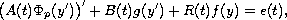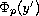Electron. J. Diff. Eqns., Vol. 2007(2007), No. 136, pp. 1-9.

### Existence of global solutions for systems of second-order differential equations with p-Laplacian Milan Medved, Eva Pekarkova

Abstract:
We obtain sufficient conditions for the existence of global solutions for the systems of differential equationswhereis the multidimensional p-Laplacian.

Submitted April 17, 2007. Published October 15, 2007.
Math Subject Classifications: 34C11.
Key Words: Second order differential equation; p-Laplacian; global solution.

Show me the PDF file (237 KB), TEX file, and other files for this article.Milan Medved Department of Mathematical Analysis and Numerical Mathematics Faculty of Mathematics, Physics and Informatics Comenius University, 842 48 Bratislava, Slovakia email: medved@fmph.uniba.sk Eva Pekárková Department of Mathematics and Statistics Faculty of Science, Masaryk University Janáckovo nám. 2a, CZ-602 00 Brno, Czech Republic email: pekarkov@math.muni.cz